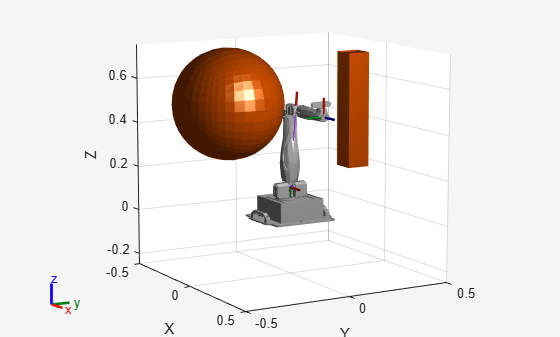# sampleGaussian

Sample state using Gaussian distribution

## Syntax

``states = sampleGaussian(manipSS,meanState,stdDev)``
``states = sampleGaussian(manipSS,meanState,stdDev,numSamples)``

## Description

example

````states = sampleGaussian(manipSS,meanState,stdDev)` samples a state in the state space `manipSS` from a Gaussian (normal) distribution centered on the mean `meanState` with the standard deviation, `stdDev`.```
````states = sampleGaussian(manipSS,meanState,stdDev,numSamples)` samples the number of multiple states specified by `numSamples`.```

## Examples

collapse all

Generate states to form a path, validate motion between states, and check for self-collisions and environmental collisions with objects in your world. The `manipulatorStateSpace` object represents the joint configuration space of your rigid body tree robot model, and can sample states, calculate distances, and enforce state bounds. The `manipulatorCollisionBodyValidator` object validates the state and motion based on the collision bodies in your robot model and any obstacles in your environment.

Use the `loadrobot` function to access predefined robot models. This example uses the Quanser QArm™ robot and joint configurations are specified as row vectors.

```robot = loadrobot("quanserQArm",DataFormat="row"); figure(Visible="on") show(robot); xlim([-0.5 0.5]) ylim([-0.5 0.5]) zlim([-0.25 0.75]) hold on```Configure State Space and State Validation

Create the state space and state validator from the robot model.

```ss = manipulatorStateSpace(robot); sv = manipulatorCollisionBodyValidator(ss,SkippedSelfCollisions="parent");```

Set the validation distance to `0.05`, which is based on the distance normal between two states. You can configure the validator to ignore self collisions to improve the speed of validation, but must consider whether your robot model has the proper joint limit settings set to ensure it does not collide with itself.

```sv.ValidationDistance = 0.05; sv.IgnoreSelfCollision = true;```

Place collision objects in the robot environment. Set the `Environment` property of the collision validator object using a cell array of objects.

```box = collisionBox(0.1,0.1,0.5); % XYZ Lengths box.Pose = trvec2tform([0.2 0.2 0.5]); % XYZ Position sphere = collisionSphere(0.25); % Radius sphere.Pose = trvec2tform([-0.2 -0.2 0.5]); % XYZ Position env = {box sphere}; sv.Environment = env;```

Visualize the environment.

```for i = 1:length(env) show(env{i}) end view(60,10)```Plan Path

Start with the home configuration as the first point on the path.

```rng(0); % Repeatable results start = homeConfiguration(robot); path = start; idx = 1;```

Plan a path using these steps, in a loop:

• Sample a nearby goal configuration, using the Gaussian distribution, by specifying the standard deviation for each joint angle.

• Check if the sampled goal state is valid.

• If the sampled goal state is valid, check if the motion between states is valid and, if so, add it to the path.

```for i = 2:25 goal = sampleGaussian(ss,start,0.25*ones(4,1)); validState = isStateValid(sv,goal); if validState % If state is valid, check motion between states. [validMotion,~] = isMotionValid(sv,path(idx,:),goal); if validMotion % If motion is valid, add to path. path = [path; goal]; idx = idx + 1; end end end```

Visualize Path

After generating the path of valid motions, visualize the robot motion. Because you sampled random states near the home configuration, you should see the arm move around that initial configuration.

To visualize the path of the end effector in 3-D, get the transformation, relative to the base world frame at each point. Store the points as an xyz translation vector. Plot the path of the end effector.

```eePose = nan(3,size(path,1)); for i = 1:size(path,1) show(robot,path(i,:),PreservePlot=false); eePos(i,:) = tform2trvec(getTransform(robot,path(i,:),"END-EFFECTOR")); % XYZ translation vector plot3(eePos(:,1),eePos(:,2),eePos(:,3),"-b",LineWidth=2) drawnow end```## Input Arguments

collapse all

Manipulator state space, specified as a `manipulatorStateSpace` object, which is a subclass of `nav.StateSpace` (Navigation Toolbox).

Mean state position, specified as an n-element row vector, where n is the dimension of the state space specified in the `NumStateVariables` property of `manipSS`.

Standard deviation around the mean state, specified as an n-element row vector. Each element corresponds to an element in `meanState`.

Number of samples, specified as a positive integer.

## Output Arguments

collapse all

Sampled states from the state space, returned as an n-element row vector or m-by-n matrix. n is the dimension of the state space specified in the `NumStateVariables` property of `manipSS`. m is the number of samples specified in `numSamples`. All states are sampled within the bounds specified by the `StateBounds` property of `manipSS`.

## Version History

Introduced in R2021b